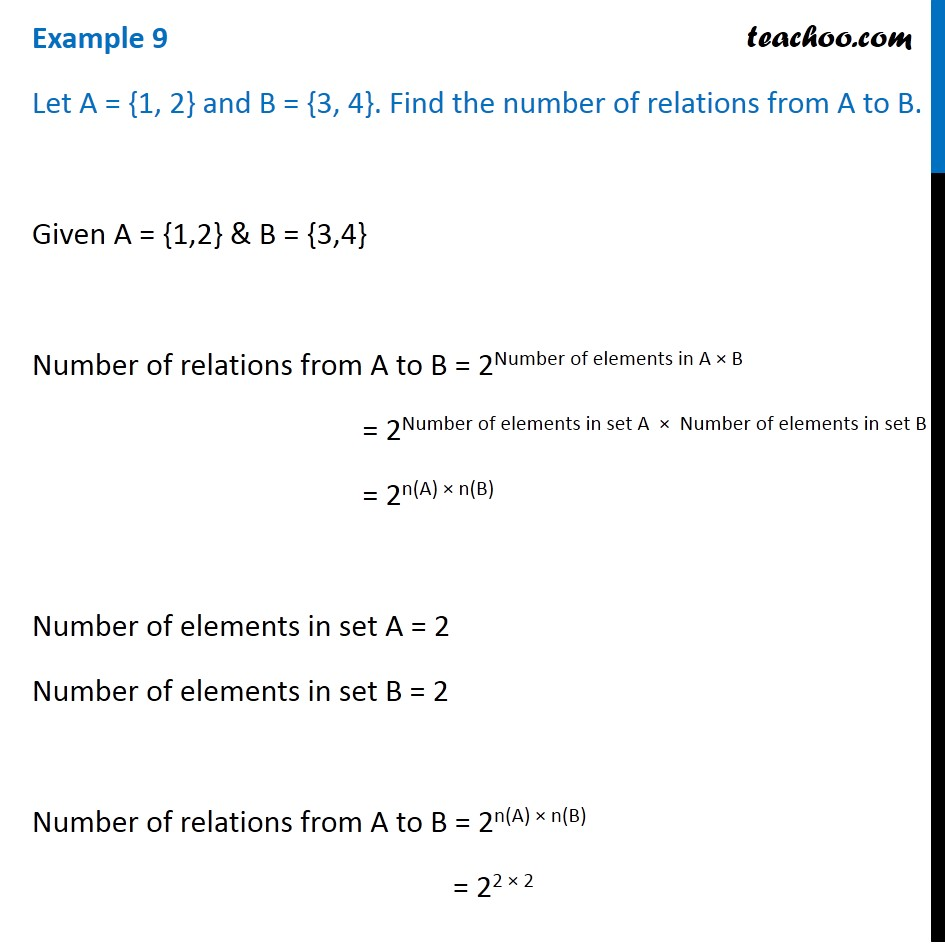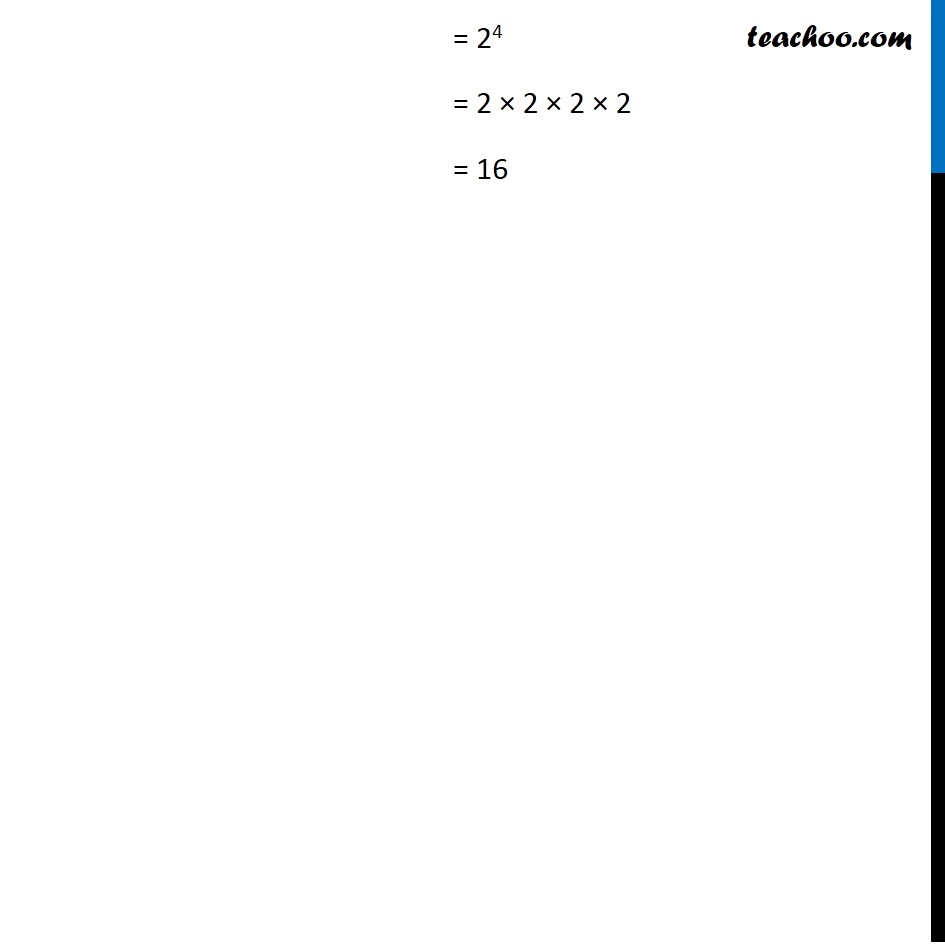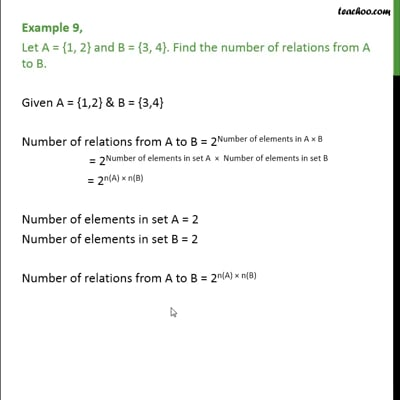Examples

Chapter 2 Class 11 Relations and Functions
Serial order wiseExample 9

Let A = {1, 2} and B = {3, 4}. Find the number of relations from A to B.

Given A = {1,2} & B = {3,4}

Number of relations from A to B = 2Number of elements in A × B

= 2Number of elements in set A  ×  Number of elements in set B

= 2n(A) × n(B)

Number of elements in set A = 2

Number of elements in set B = 2

Number of relations from A to B = 2n(A) × n(B)

= 22 × 2This video is only available for Teachoo black users

Maths Crash Course - Live lectures + all videos + Real time Doubt solving!

### Transcript

Example 9 Let A = {1, 2} and B = {3, 4}. Find the number of relations from A to B. Given A = {1,2} & B = {3,4} Number of relations from A to B = 2Number of elements in A × B = 2Number of elements in set A × Number of elements in set B = 2n(A) × n(B) Number of elements in set A = 2 Number of elements in set B = 2 Number of relations from A to B = 2n(A) × n(B) = 22 × 2 = 24 = 2 × 2 × 2 × 2 = 16